# Introduction

The R Bayesian evidence synthesis Tools (RBesT) have been created to facilitate the use of historical information in clinical trials. Once relevant historical information has been identified, RBesT supports the derivation of informative priors via the Meta-Analytic-Predictive (MAP) approach , the evaluation of the trial’s operating characteristics, and the data analysis with the actual trial data. RBesT has been developed for endpoints from a number of well known distributions. Here we consider an example for a normally distributed response variable.

# Trial Design with Historical Control Data

## Historical Data

Let’s look at the Crohn’s disease example data  (data-set crohn in RBesT). The primary endpoint is the change from baseline in Crohn’s Disease Activity Index (CDAI), which is assumed to be normally distributed. Note that for CDAI, an improved outcome corresponds to a negative change from baseline.

First from historical studies we get the estimated standard deviation of the response variable as $$\sigma$$ = 88, which is used to obtain the standard errors of the effect estimates.

dat <- crohn
crohn_sigma <- 88
dat$y.se <- crohn_sigma/sqrt(dat$n)
study n y y.se
Gastr06 74 -51 10.229792
AIMed07 166 -49 6.830125
NEJM07 328 -36 4.858987
Gastr01a 20 -47 19.677398
APhTh04 25 -90 17.600000
Gastr01b 58 -54 11.554966

## Derivation of MAP Prior

The MAP prior can be derived with the function gMAP. The between-trial heterogeneity parameter $$\tau$$ governs how much information will be shared from the historical trials into the design and analysis of the future trials. In the normal case with a known sampling standard deviation $$\sigma$$, the amount of borrowing from historical data depends on the ratio $$\tau/\sigma$$. A conservative choice for the prior on $$\tau$$ is a HalfNormal(0, $$\sigma/2$$) distribution. For the prior on the intercept we recommend a “unit-information” prior  which is set to a precision corresponding to a single observation and centered here at no change from baseline. Please refer to the help page ?gMAP for detailed information. The set.seed function is used to make the results exactly reproducible.

library(RBesT)
set.seed(1234)
map_mcmc <- gMAP(cbind(y, y.se) ~ 1 | study,
weights=n,data=dat,
family=gaussian,
beta.prior=cbind(0, crohn_sigma),
tau.dist="HalfNormal",tau.prior=cbind(0,crohn_sigma/2))
print(map_mcmc)
## Generalized Meta Analytic Predictive Prior Analysis
##
## Call:  gMAP(formula = cbind(y, y.se) ~ 1 | study, family = gaussian,
##     data = dat, weights = n, tau.dist = "HalfNormal", tau.prior = cbind(0,
##         crohn_sigma/2), beta.prior = cbind(0, crohn_sigma))
##
## Exchangeability tau strata: 1
## Prediction tau stratum    : 1
## Maximal Rhat              : 1
## Estimated reference scale : 88
##
## Between-trial heterogeneity of tau prediction stratum
##  mean    sd  2.5%   50% 97.5%
## 14.40  9.68  1.37 12.50 37.80
##
## MAP Prior MCMC sample
##  mean    sd  2.5%   50% 97.5%
## -49.8  19.3 -91.5 -48.5 -11.4
## a graphical representation is also available
pl <- plot(map_mcmc)

## a number of plots are immediately defined
names(pl)
##  "densityThetaStar"     "densityThetaStarLink" "forest_model"
## forest plot with model estimates
print(pl$forest_model)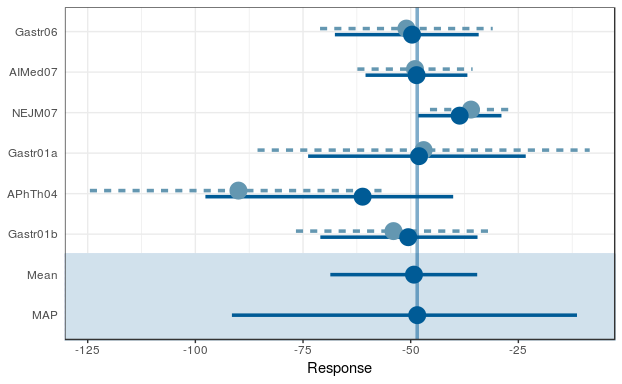## Approximation of MAP Prior using a Mixture Distribution Next, the MCMC MAP prior from the previous section is converted to a parametric representation with the automixfit function. This function fits a parametric mixture representation using expectation-maximization (EM). The number of mixture components is chosen automatically using AIC. One can also specify the number of components for the mixture via mixfit function and compare with the automixfit outcome. map <- automixfit(map_mcmc) print(map) ## EM for Normal Mixture Model ## Log-Likelihood = -17088.79 ## ## Univariate normal mixture ## Reference scale: 88 ## Mixture Components: ## comp1 comp2 comp3 comp4 ## w 0.445234009 0.323869406 0.228678186 0.002218398 ## m -50.818522717 -46.646210971 -52.165606465 -56.943273786 ## s 15.561155292 6.972499085 30.812700200 111.492236412 ## check accuracy of mixture fit plot(map)$mix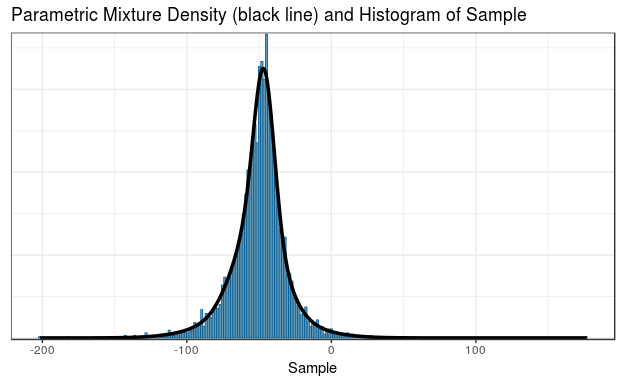## choose the number of components manually
map_2 <- mixfit(map_mcmc, Nc = 2)
print(map_2)
## EM for Normal Mixture Model
## Log-Likelihood = -17137.46
##
## Univariate normal mixture
## Reference scale: 88
## Mixture Components:
##   comp1       comp2
## w   0.6499501   0.3500499
## m -48.0554251 -53.0074311
## s  10.1482561  29.3142498
plot(map_2)\$mix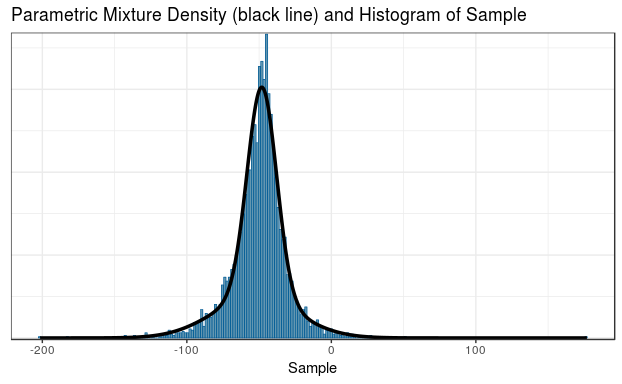## Effective Sample Size (ESS)

The main advantage of using historical information is the possibility to reduce the number of control patients, as the informative prior is effectively equivalent to a certain number of control patients. This is called the effective sample size (ESS) and can be calculated in RBesT with the ess function.

Note, however, that various methods to calculate ESS have been proposed, and that they can give fairly different answers. The default moment-based approach yields conservative ESS, while the Morita et. al  approach normally gives an over optimistic ESS. From experience, a realistic ESS can be obtained by applying the Morita et. al method to a MAP approximation with few mixture components.

In the study protocol of this Crohn’s disease data example, the moment-based ESS of 20 was used to reduce the planned sample size of the control group.

ess(map)
## Using default prior reference scale 88
##  21
ess(map,   method="morita")
## Using default prior reference scale 88
##  101
ess(map_2, method="morita")
## Using default prior reference scale 88
##  65

## Robustification of MAP Prior

We recommend robustifying  the prior with the robustify function, which protects against type-I error inflation in presence of prior-data conflict. For the normal case we strongly recommend explicitly choosing the mean of the robust component. We use $$-50$$ consistent with the mean of the MAP prior. Furthermore, 20% probability is used for the additional robust (unit-information) mixture component. The choice of such probability reflects the confidence about the validitiy of the model assumptions, i.e. the possibility of a non-exchangable control group to be enrolled per inclusion/exclusion criteria in the current trial as compared to the historical control group population. Note that robustification decreases the ESS (numbers not shown).

## add a 20% non-informative mixture component
map_robust <- robustify(map, weight=0.2, mean=-50)
## Using default prior reference scale 88
print(map_robust)
## Univariate normal mixture
## Reference scale: 88
## Mixture Components:
##   comp1         comp2         comp3         comp4         robust
## w   0.356187207   0.259095525   0.182942549   0.001774718   0.200000000
## m -50.818522717 -46.646210971 -52.165606465 -56.943273786 -50.000000000
## s  15.561155292   6.972499085  30.812700200 111.492236412  88.000000000

## Operating Characteristics of Design Options

Typically, operating characteristics are required to evaluate the proposed design or compare a few design options. RBesT requires the input of decision rules via decision2S function and then calculates the operating characteristics with the oc2S function. The calculation is expedited based on analytic expressions. In the following we compare a few design options, which differ in the choice of the control priors and the sample size of the control group for the planned trial. There may be other design factors (such as the outcome standard deviation), which should be considered when comparing design options. Such factors are not considered here for simplicity purpose.

### Decision Rules

Consider this 2-arm design of placebo (with an informative prior) against an experimental treatment. The dual-criterion for success is defined as follows: \begin{align*} \textrm{Criterion 1:} & \Pr(y_{act} - y_{pbo} \lt 0) &> 0.95 \\ \textrm{Criterion 2:} & \Pr(y_{act} - y_{pbo} \lt -50) &> 0.50. \end{align*}

Equivalently, the second criterion requires that the posterior median difference exceeds -50. The dual-criteria account for statistical significance as well as clinical relevance. Note that a negative change from baseline in CDAI corresponds to improvement.

## dual decision criteria
## pay attention to "lower.tail" argument and the order of active and pbo
poc <- decision2S(pc=c(0.95,0.5), qc=c(0,-50), lower.tail=TRUE)
print(poc)
## 2 sample decision function
## Conditions for acceptance:
## P(x1 - x2 <= 0) > 0.95
## P(x1 - x2 <= -50) > 0.5
## Link: identity

### Design Options

For the active group we use the same weakly informative (unit-information) prior as used in the robustification step of the MAP prior. Also, we set up the few design options with different choices of control prior and different sizes of control group.

## set up prior for active group
weak_prior <- mixnorm(c(1,-50,1), sigma=crohn_sigma, param = 'mn')
n_act <- 40
n_pbo <- 20

## four designs
## "b" means a balanced design, 1:1
## "ub" means 40 in active and 20 in placebo
design_noprior_b  <- oc2S(weak_prior, weak_prior, n_act, n_act, poc,
sigma1=crohn_sigma, sigma2=crohn_sigma)
design_noprior_ub <- oc2S(weak_prior, weak_prior, n_act, n_pbo, poc,
sigma1=crohn_sigma, sigma2=crohn_sigma)
design_nonrob_ub  <- oc2S(weak_prior, map, n_act, n_pbo, poc,
sigma1=crohn_sigma, sigma2=crohn_sigma)
design_rob_ub     <- oc2S(weak_prior, map_robust, n_act, n_pbo, poc,
sigma1=crohn_sigma, sigma2=crohn_sigma)

### Type I Error

The type I can be increased compared to the nominal $$\alpha$$ level in case of a conflict between the trial data and the prior. The robustified MAP prior can reduce the type I error inflation in this case to a lower level.

# the range for true values
cfb_truth <- seq(-120, -40, by=1)

typeI1 <- design_noprior_b(cfb_truth, cfb_truth)
typeI2 <- design_noprior_ub(cfb_truth, cfb_truth)
typeI3 <- design_nonrob_ub(cfb_truth, cfb_truth)
typeI4 <- design_rob_ub(cfb_truth, cfb_truth)

ocI <- rbind(data.frame(cfb_truth=cfb_truth, typeI=typeI1,
design="40:40 with non-informative priors"),
data.frame(cfb_truth=cfb_truth, typeI=typeI2,
design="40:20 with non-informative priors"),
data.frame(cfb_truth=cfb_truth, typeI=typeI3,
design="40:20 with non-robust prior for placebo"),
data.frame(cfb_truth=cfb_truth, typeI=typeI4,
design="40:20 with robust prior for placebo")
)
qplot(cfb_truth, typeI, data=ocI, colour=design, geom="line", main="Type I Error") +
xlab(expression(paste('True value of change from baseline ', mu[act] == mu[pbo]))) +
ylab('Type I error') +
coord_cartesian(ylim=c(0,0.2)) +
theme(legend.justification=c(1,1),legend.position=c(0.95,0.85))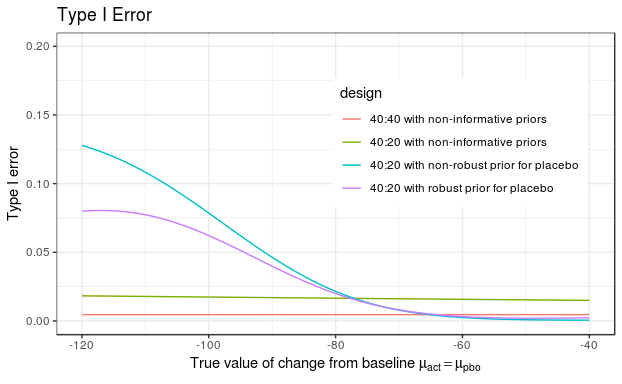### Power

The power shows the gain of using an informative prior for the control arm; i.e. 90% power is reached for smaller $$\delta$$ values compared to the design with weakly informative priors for both arms or the balanced design.

delta <- seq(-80,0,by=1)
m <- summary(map)["mean"]
cfb_truth1 <- m + delta   # active for 1
cfb_truth2 <- m + 0*delta # pbo for 2

power1 <- design_noprior_b(cfb_truth1, cfb_truth2)
power2 <- design_noprior_ub(cfb_truth1, cfb_truth2)
power3 <- design_nonrob_ub(cfb_truth1, cfb_truth2)
power4 <- design_rob_ub(cfb_truth1, cfb_truth2)

ocP <- rbind(data.frame(cfb_truth1=cfb_truth1, cfb_truth2=cfb_truth2,
delta=delta, power=power1,
design="40:40 with non-informative priors"),
data.frame(cfb_truth1=cfb_truth1, cfb_truth2=cfb_truth2,
delta=delta, power=power2,
design="40:20 with non-informative priors"),
data.frame(cfb_truth1=cfb_truth1, cfb_truth2=cfb_truth2,
delta=delta, power=power3,
design="40:20 with non-robust prior for placebo"),
data.frame(cfb_truth1=cfb_truth1, cfb_truth2=cfb_truth2,
delta=delta, power=power4,
design="40:20 with robust prior for placebo")
)

qplot(delta, power, data=ocP, colour=design, geom="line", main="Power") +
xlab('True value of difference (act - pbo)')+ ylab('Power') +
scale_y_continuous(breaks=c(seq(0,1,0.2),0.9)) +
scale_x_continuous(breaks=c(seq(-80,0,20),-70)) +
geom_hline(yintercept=0.9,linetype=2) +
geom_vline(xintercept=-70,linetype=2) +
theme(legend.justification=c(1,1),legend.position=c(0.95,0.85))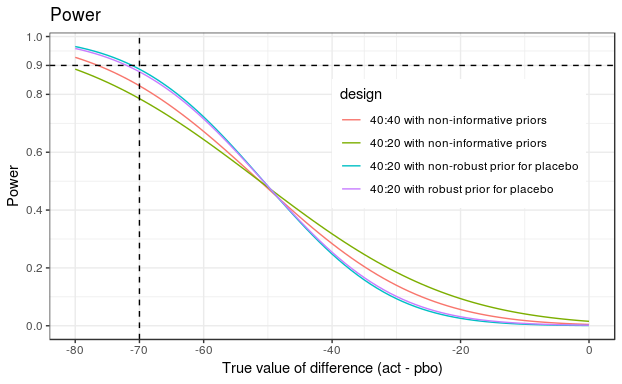# Final Analysis with Trial Data

When the actual trial data are available, the final analysis can be run with RBesT via the postmix function. The real data are used for this example, where the trial data led to a negative conclusion. However, note that postmix assumes that the sampling standard deviation is known and fixed. Therefore, no uncertainty in its estimate is taken into account.

## one can either use summary data or individual data. See ?postmix.
y.act <- -29.15
y.act.se <- 16.69
n.act <- 39

y.pbo <- -76.01
y.pbo.se <- 21.93
n.pbo <- 20

## first obtain posterior distributions
post_act <- postmix(weak_prior, m=y.act, se=y.act.se)
post_pbo <- postmix(map_robust, m=y.pbo, se=y.pbo.se)

## then calculate probability for the dual criteria
## and compare to the predefined threshold values
p1 <- pmixdiff(post_act, post_pbo, 0); print(p1)
##  0.08548332
p2 <- pmixdiff(post_act, post_pbo, -50); print(p2)
##  9.052265e-05
print(p1>0.95 & p2>0.5)
##  FALSE
## or we can use the decision function
poc(post_act, post_pbo)
##  0

### R Session Info

sessionInfo()
## R version 3.5.1 (2018-07-02)
## Platform: x86_64-pc-linux-gnu (64-bit)
## Running under: Red Hat Enterprise Linux
##
## Matrix products: default
## BLAS/LAPACK: /CHBS/apps/busdev_apps/eb/software/imkl/2018.3.222-GCC-6.4.0-2.28/compilers_and_libraries_2018.3.222/linux/mkl/lib/intel64_lin/libmkl_gf_lp64.so
##
## locale:
##   LC_CTYPE=en_US.UTF-8       LC_NUMERIC=C
##   LC_TIME=en_US.UTF-8        LC_COLLATE=C
##   LC_MONETARY=en_US.UTF-8    LC_MESSAGES=en_US.UTF-8
##   LC_PAPER=en_US.UTF-8       LC_NAME=C
##   LC_ADDRESS=C               LC_TELEPHONE=C
##  LC_MEASUREMENT=en_US.UTF-8 LC_IDENTIFICATION=C
##
## attached base packages:
##  stats     graphics  grDevices utils     datasets  methods   base
##
## other attached packages:
##   forcats_0.3.0   stringr_1.3.1   readr_1.1.1     tidyr_0.8.1
##   tibble_1.4.2    tidyverse_1.2.1 scales_1.0.0    bindrcpp_0.2.2
##   ggplot2_3.1.0   purrr_0.2.5     dplyr_0.7.6     bayesplot_1.6.0
##  knitr_1.20      RBesT_1.3-7     Rcpp_0.12.19
##
## loaded via a namespace (and not attached):
##   lubridate_1.7.4    mvtnorm_1.0-8      lattice_0.20-35
##   prettyunits_1.0.2  assertthat_0.2.0   rprojroot_1.3-2
##   digest_0.6.15      cellranger_1.1.0   R6_2.2.2
##  plyr_1.8.4         ggridges_0.5.0     backports_1.1.2
##  stats4_3.5.1       evaluate_0.11      httr_1.3.1
##  highr_0.7          pillar_1.3.0       rlang_0.2.1
##  readxl_1.1.0       lazyeval_0.2.1     rstudioapi_0.7
##  callr_2.0.4        checkmate_1.8.5    rmarkdown_1.10
##  labeling_0.3       loo_2.0.0          munsell_0.5.0
##  broom_0.5.0        modelr_0.1.2       compiler_3.5.1
##  rstan_2.18.2       pkgconfig_2.0.1    pkgbuild_1.0.2
##  htmltools_0.3.6    tidyselect_0.2.4   gridExtra_2.3
##  codetools_0.2-15   matrixStats_0.54.0 crayon_1.3.4
##  withr_2.1.2        grid_3.5.1         nlme_3.1-137
##  jsonlite_1.5       gtable_0.2.0       magrittr_1.5
##  StanHeaders_2.18.0 cli_1.0.0          stringi_1.2.4
##  debugme_1.1.0      reshape2_1.4.3     xml2_1.2.0
##  Formula_1.2-3      tools_3.5.1        glue_1.3.0
##  hms_0.4.2          processx_3.1.0     parallel_3.5.1
##  yaml_2.2.0         inline_0.3.15      colorspace_1.3-2
##  rvest_0.3.2        bindr_0.1.1        haven_1.1.2